# Existence of the solutions for a class of nonlinear fractional order three-point boundary value problems with resonance

## Abstract

A class of nonlinear fractional order differential equation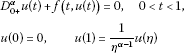is investigated in this paper, where ${D}_{0+}^{\alpha }$ is the standard Riemann-Liouville fractional derivative of order $1<\alpha \le 2$, $0<\eta <1$, $f\in C\left(\left[0,1\right]×R,R\right)$. Using intermediate value theorem, we obtain a sufficient condition for the existence of the solutions for the above fractional order differential equations.

## 1 Introduction

Consider the following boundary value problem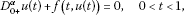(1.1)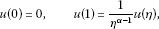(1.2)

where ${D}_{0+}^{\alpha }$ is the standard Riemann-Liouville fractional derivative of order $1<\alpha \le 2$, $0<\eta <1$ and $f\in C\left(\left[0,1\right]×R,R\right)$.

In the last few decades, many authors have investigated fractional differential equations which have been applied in many fields such as physics, mechanics, chemistry, engineering etc. (see references [1, 6, 10, 2123]). Especially, many works have been devoted to the study of initial value problems and bounded value problems for fractional order differential equations [12, 13, 15, 24].

Recently, the existence of positive solutions of fractional differential equations has attracted many authors’ attention [25, 8, 9, 12, 14, 1720, 25, 26]. Using some fixed point theorems, they obtained some nice existence conditions for positive solutions.

In more recent works, Jiang and Yuan  considered the following boundary value problem of fractional differential equations(1.3)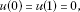(1.4)

where ${D}_{0+}^{\alpha }$ is the standard Riemann-Liouville fractional derivative of order $1<\alpha <2$ and $f:\left[0,1\right]×{R}_{+}\to {R}_{+}$ is continuous. Using some properties of the Green function $G\left(t,s\right)$, they obtain some new sufficient conditions for the existence of positive solutions for the above problem.

Further, Li, Luo, and Zhou  investigated the following fractional order three-point boundary value problems(1.5)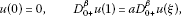(1.6)

where ${D}_{0+}^{\alpha }$ is the standard Riemann-Liouville fractional derivative of order $1<\alpha \le 2$$0\le \beta \le 1$$0\le a\le 1$$\xi \in \left(0,1\right)$$a{\xi }^{\alpha -\beta -2}\le 1-\beta$$0\le \alpha -\beta -1$, and $f:\left[0,1\right]×{R}_{+}\to {R}_{+}$ is continuous.

In this paper, we discuss the boundary value problem (1.1)-(1.2). Using some properties of the Green function $G\left(t,s\right)$ and intermediate value theorem, we establish some sufficient conditions for the existence of the positive solutions of the problem (1.1)-(1.2).

The paper is arranged as follows: In Section 2, we introduce some definitions for fractional order differential equations and give our main results for the boundary value problem (1.1)-(1.2). We give some lemmas for our results in Section 3. In Section 4, we prove our main result; and finally, we give an example to illustrate our results.

## 2 Main results

In this section, we introduce some definitions and preliminary facts which are used in this paper.

Definition 2.1 ([1, 10])

The fractional integral of order α with the lower limit ${t}_{0}$ for a function f is defined as

${I}_{{t}_{0}+}^{\alpha }\left(f\left(t\right)\right)=\frac{1}{\mathrm{\Gamma }\left(\alpha \right)}{\int }_{{t}_{0}}^{t}\frac{f\left(s\right)}{{\left(t-s\right)}^{1-\alpha }}\phantom{\rule{0.2em}{0ex}}ds,\phantom{\rule{1em}{0ex}}t>{t}_{0},\alpha >0,$

provided that the integral on the right-hand side is point-wise defined on $\left[{t}_{0},\mathrm{\infty }\right)$, where Γ is the Gamma function.

Definition 2.2 ([1, 10])

Riemann-Liouville derivative of order α with the lower limit ${t}_{0}$ for a function $f:\left[0,\mathrm{\infty }\right)\to R$ can be written as

${D}_{{t}_{0}+}^{\alpha }\left(f\left(t\right)\right)=\frac{1}{\mathrm{\Gamma }\left(n-\alpha \right)}\frac{{d}^{n}}{d{t}^{n}}{\int }_{{t}_{0}}^{t}\frac{f\left(s\right)}{{\left(t-s\right)}^{\alpha +1-n}}\phantom{\rule{0.2em}{0ex}}ds,\phantom{\rule{1em}{0ex}}t>{t}_{0},n-1<\alpha \le n,$

where n is a positive integer.

We call the function $u\left(t\right)$ a solution of (1.1)-(1.2) if $u\left(t\right)\in C\left[0,1\right]\cap L\left[0,1\right]$ with a fractional derivative of order α belongs to $C\left[0,1\right]\cap L\left[0,1\right]$ and satisfies Equation (1.1) and the boundary condition (1.2).

We also need to introduce some lemmas as follows, which will be used in the proof of our main theorems.

Lemma 2.1 ()

Assume that$h\left(t\right)\in C\left(0,1\right)\cap L\left(0,1\right)$with a fractional derivative of order$\alpha >0$belongs to$C\left(0,1\right)\cap L\left(0,1\right)$. Then, the fractional equation

${D}_{{t}_{0}+}^{\alpha }\left(h\left(t\right)\right)=0$
(2.1)

has solutions

$h\left(t\right)={c}_{1}{t}^{\alpha -1}+{c}_{2}{t}^{\alpha -2}+\cdots +{c}_{n}{t}^{\alpha -n},\phantom{\rule{1em}{0ex}}{c}_{i}\in R,i=1,2,\dots ,n,n=\left[\alpha \right]+1.$
(2.2)

Lemma 2.2 ()

Assume that$h\left(t\right)\in C\left(0,1\right)\cap L\left(0,1\right)$with a fractional derivative of order$\alpha >0$belongs to$C\left(0,1\right)\cap L\left(0,1\right)$. Then

${I}_{{t}_{0}+}^{\alpha }{D}_{{t}_{0}+}^{\alpha }h\left(t\right)=h\left(t\right)+{c}_{1}{t}^{\alpha -1}+{c}_{2}{t}^{\alpha -2}+\cdots +{c}_{n}{t}^{\alpha -n}$
(2.3)

for some${c}_{i}\in R$, $i=1,2,\dots ,n$, $n=\left[\alpha \right]+1$.

Lemma 2.3 ()

Suppose that X be a Banach space, $C\subset X$is closed and convex. Assume that U is a relatively open subset of C with$0\in U$, and$T:\overline{U}\to C$is a completely continuous operator. Then, either

1. (i)

T has a fixed point in $\overline{U}$, or

2. (ii)

there exist $u\in \partial U$ and $\gamma \in \left(0,1\right)$ with $u=\gamma Tu$.

Throughout this paper, we assume that $f\left(t,u\right)$ satisfies the following:

(H)$f\left(t,u\right)\in C\left(\left[0,1\right]×R,R\right)$, and there exist two positive functions$a\left(t\right)\in C\left(\left[0,1\right],{R}_{+}\right)$and$b\left(t\right)\in C\left(\left[0,1\right],{R}_{+}\right)$such that

$|f\left(t,{t}^{\alpha -1}u\right)|\le a\left(t\right)+b\left(t\right){|u|}^{p},\phantom{\rule{1em}{0ex}}t\in \left[0,1\right],$
(2.4)

where$0\le p\le 1$. Furthermore,

$\underset{u\to ±\mathrm{\infty }}{lim}f\left(t,{t}^{\alpha -1}u\right)=±\mathrm{\infty }$
(2.5)

for any$t\in \left(0,1\right)$.

We have our main results:

Theorem 2.1 Suppose that (H) holds. If

${\int }_{0}^{1}{G}^{\ast }\left(s,s\right)b\left(s\right)\phantom{\rule{0.2em}{0ex}}ds<1,$
(2.6)

then the boundary value problem (1.1)-(1.2) has at least one solution, where

${G}^{\ast }\left(s,s\right)=\frac{1}{\mathrm{\Gamma }\left(\alpha \right)\left(1-{\eta }^{\alpha -1}\right)}\left\{\begin{array}{cc}{\left(1-s\right)}^{\alpha -1}-{\left(\eta -s\right)}^{\alpha -1},\hfill & 0\le s\le \eta ,\hfill \\ {\left(1-s\right)}^{\alpha -1},\phantom{\rule{1em}{0ex}}\eta \le s\le 1.\hfill \end{array}$

## 3 Some lemmas

Let $\mathrm{\Omega }=C\left[0,1\right]$, $u\in \mathrm{\Omega }$ equipped the norm

$\parallel u\parallel =\underset{0\le t\le 1}{sup}|u\left(t\right)|,$
(3.1)

then Ω is a Banach space.

We first give some lemmas as follows:

Lemma 3.1 Problem (1.1)-(1.2) is equivalent to the following integral equation

$u\left(t\right)={\int }_{0}^{1}G\left(t,s\right)f\left(s,u\left(s\right)\right)\phantom{\rule{0.2em}{0ex}}ds+u\left(1\right){t}^{\alpha -1},$
(3.2)

where

$G\left(t,s\right)=\left\{\begin{array}{c}\frac{{t}^{\alpha -1}{\left(1-s\right)}^{\alpha -1}-{t}^{\alpha -1}{\left(\eta -s\right)}^{\alpha -1}-\left(1-{\eta }^{\alpha -1}\right){\left(t-s\right)}^{\alpha -1}}{\mathrm{\Gamma }\left(\alpha \right)\left(1-{\eta }^{\alpha -1}\right)},\hfill \\ \phantom{\rule{1em}{0ex}}0\le s\le min\left\{t,\eta \right\}\le 1;\hfill \\ \frac{{t}^{\alpha -1}{\left(1-s\right)}^{\alpha -1}-{t}^{\alpha -1}{\left(\eta -s\right)}^{\alpha -1}}{\mathrm{\Gamma }\left(\alpha \right)\left(1-{\eta }^{\alpha -1}\right)},\phantom{\rule{1em}{0ex}}0\le t\le s\le \eta \le 1;\hfill \\ \frac{{t}^{\alpha -1}{\left(1-s\right)}^{\alpha -1}-\left(1-{\eta }^{\alpha -1}\right){\left(t-s\right)}^{\alpha -1}}{\mathrm{\Gamma }\left(\alpha \right)\left(1-{\eta }^{\alpha -1}\right)},\phantom{\rule{1em}{0ex}}0\le \eta \le s\le t\le 1;\hfill \\ \frac{{t}^{\alpha -1}{\left(1-s\right)}^{\alpha -1}}{\mathrm{\Gamma }\left(\alpha \right)\left(1-{\eta }^{\alpha -1}\right)},\phantom{\rule{1em}{0ex}}0\le max\left\{t,\eta \right\}\le s\le 1.\hfill \end{array}$
(3.3)

Proof The sufficiency is obvious, we only need to prove the necessity.

Suppose that $u\left(t\right)$ is a solution of the problem (1.1)-(1.2). Integrating both sides of (1.1) of α order from 0 to t with respect to t, it follows that

$u\left(t\right)=-\frac{1}{\mathrm{\Gamma }\left(\alpha \right)}{\int }_{0}^{t}{\left(t-s\right)}^{\alpha -1}f\left(s,u\left(s\right)\right)\phantom{\rule{0.2em}{0ex}}ds+{c}_{1}{t}^{\alpha -1}+{c}_{2}{t}^{\alpha -2}.$
(3.4)

According to (1.2) and (3.4), we have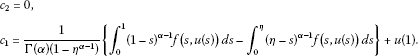(3.5)

Combining (3.4) and (3.5), we obtain

$\begin{array}{rcl}u\left(t\right)& =& -\frac{1}{\mathrm{\Gamma }\left(\alpha \right)\left(1-{\eta }^{\alpha -1}\right)}{\int }_{0}^{t}\left(1-{\eta }^{\alpha -1}\right){\left(t-s\right)}^{\alpha -1}f\left(s,u\left(s\right)\right)\phantom{\rule{0.2em}{0ex}}ds\\ +\frac{1}{\mathrm{\Gamma }\left(\alpha \right)\left(1-{\eta }^{\alpha -1}\right)}\left\{{\int }_{0}^{1}{t}^{\alpha -1}{\left(1-s\right)}^{\alpha -1}f\left(s,u\left(s\right)\right)\phantom{\rule{0.2em}{0ex}}ds-{\int }_{0}^{\eta }{t}^{\alpha -1}{\left(\eta -s\right)}^{\alpha -1}f\left(s,u\left(s\right)\right)\phantom{\rule{0.2em}{0ex}}ds\right\}\\ +u\left(1\right){t}^{\alpha -1}.\end{array}$

According to (3.3), it is easy to show that (3.2) holds. The proof is completed. □

Lemma 3.2 For any$\left(t,s\right)\in \left[0,1\right]×\left[0,1\right]$, $G\left(t,s\right)$is continuous, and$G\left(t,s\right)>0$for any$\left(t,s\right)\in \left(0,1\right)×\left(0,1\right)$.

Proof The continuity of $G\left(t,s\right)$ for $\left(t,s\right)\in \left[0,1\right]×\left[0,1\right]$ is obvious.

Let

${g}_{1}\left(t,s\right)={t}^{\alpha -1}{\left(1-s\right)}^{\alpha -1}-{t}^{\alpha -1}{\left(\eta -s\right)}^{\alpha -1}-\left(1-{\eta }^{\alpha -1}\right){\left(t-s\right)}^{\alpha -1},$

we only need to show that ${g}_{1}\left(t,s\right)>0$ for $0\le s\le min\left\{t,\eta \right\}\le 1$, the rest of the proof is similar or obvious. From the definition of ${g}_{1}\left(t,s\right)$, we have

$\begin{array}{rcl}{g}_{1}\left(t,s\right)& =& {t}^{\alpha -1}\left\{{\left(1-s\right)}^{\alpha -1}-{\left(\eta -s\right)}^{\alpha -1}-\left(1-{\eta }^{\alpha -1}\right){\left(1-\frac{s}{t}\right)}^{\alpha -1}\right\}\\ \ge & {t}^{\alpha -1}\left\{{\left(1-s\right)}^{\alpha -1}-{\left(\eta -s\right)}^{\alpha -1}-\left(1-{\eta }^{\alpha -1}\right){\left(1-s\right)}^{\alpha -1}\right\}\\ \ge & {t}^{\alpha -1}\left\{{\eta }^{\alpha -1}{\left(1-s\right)}^{\alpha -1}-{\left(\eta -s\right)}^{\alpha -1}\right\}\\ \ge & {t}^{\alpha -1}\left\{{\left(\eta -\eta s\right)}^{\alpha -1}-{\left(\eta -s\right)}^{\alpha -1}\right\}>0\end{array}$

for $0\le s\le min\left\{t,\eta \right\}\le 1$. The proof is completed. □

Let

$G\left(t,s\right)={t}^{\alpha -1}{G}^{\ast }\left(t,s\right),$

then

${G}^{\ast }\left(t,s\right)=\left\{\begin{array}{c}\frac{{\left(1-s\right)}^{\alpha -1}-{\left(\eta -s\right)}^{\alpha -1}-\left(1-{\eta }^{\alpha -1}\right){\left(1-\frac{s}{t}\right)}^{\alpha -1}}{\mathrm{\Gamma }\left(\alpha \right)\left(1-{\eta }^{\alpha -1}\right)},\hfill \\ \phantom{\rule{1em}{0ex}}0\le s\le min\left\{t,\eta \right\}\le 1;\hfill \\ \frac{{\left(1-s\right)}^{\alpha -1}-{\left(\eta -s\right)}^{\alpha -1}}{\mathrm{\Gamma }\left(\alpha \right)\left(1-{\eta }^{\alpha -1}\right)},\phantom{\rule{1em}{0ex}}0\le t\le s\le \eta \le 1;\hfill \\ \frac{{\left(1-s\right)}^{\alpha -1}-\left(1-{\eta }^{\alpha -1}\right){\left(1-\frac{s}{t}\right)}^{\alpha -1}}{\mathrm{\Gamma }\left(\alpha \right)\left(1-{\eta }^{\alpha -1}\right)}\phantom{\rule{1em}{0ex}}0\le \eta \le s\le t\le 1;\hfill \\ \frac{{\left(1-s\right)}^{\alpha -1}}{\mathrm{\Gamma }\left(\alpha \right)\left(1-{\eta }^{\alpha -1}\right)},\phantom{\rule{1em}{0ex}}0\le max\left\{t,\eta \right\}\le s\le 1.\hfill \end{array}$
(3.6)

The new Green’s function ${G}^{\ast }\left(t,s\right)$ has the following properties:

Lemma 3.3${G}^{\ast }\left(t,s\right)$is continuous for$\left(t,s\right)\in \left(0,1\right)×\left(0,1\right)$, and

$\underset{t\to 0}{lim}{G}^{\ast }\left(t,s\right):={G}^{\ast }\left(0,s\right)=\left\{\begin{array}{c}\frac{1}{\mathrm{\Gamma }\left(\alpha \right)\left(1-{\eta }^{\alpha -1}\right)}\left\{{\left(1-s\right)}^{\alpha -1}-{\left(\eta -s\right)}^{\alpha -1}\right\},\phantom{\rule{1em}{0ex}}0\le s\le \eta ,\hfill \\ \frac{1}{\mathrm{\Gamma }\left(\alpha \right)\left(1-{\eta }^{\alpha -1}\right)}{\left(1-s\right)}^{\alpha -1},\phantom{\rule{1em}{0ex}}\eta \le s\le 1.\hfill \end{array}$

Furthermore, ${G}^{\ast }\left(t,s\right)>0$for$\left(t,s\right)\in \left(0,1\right)×\left(0,1\right)$.

Lemma 3.4 For any$s\in \left(0,1\right)$, ${G}^{\ast }\left(t,s\right)$is nonincreasing with respect to$t\in \left[0,1\right]$. Especially, for any$s\in \left[0,1\right]$, $\frac{\partial {G}^{\ast }}{\partial t}\le 0$for$t\in \left[s,1\right]$, and$\frac{\partial {G}^{\ast }}{\partial t}=0$for$t\in \left[0,s\right]$. That is${G}^{\ast }\left(1,s\right)\le {G}^{\ast }\left(t,s\right)\le {G}^{\ast }\left(s,s\right)$, where

${G}^{\ast }\left(1,s\right)=\frac{1}{\mathrm{\Gamma }\left(\alpha \right)\left(1-{\eta }^{\alpha -1}\right)}\left\{\begin{array}{c}{\eta }^{\alpha -1}{\left(1-s\right)}^{\alpha -1}-{\left(\eta -s\right)}^{\alpha -1},\phantom{\rule{1em}{0ex}}0\le s\le \eta ,\hfill \\ {\eta }^{\alpha -1}{\left(1-s\right)}^{\alpha -1},\phantom{\rule{1em}{0ex}}\eta \le s\le 1,\hfill \end{array}$
(3.7)

and

${G}^{\ast }\left(s,s\right)=\frac{1}{\mathrm{\Gamma }\left(\alpha \right)\left(1-{\eta }^{\alpha -1}\right)}\left\{\begin{array}{c}{\left(1-s\right)}^{\alpha -1}-{\left(\eta -s\right)}^{\alpha -1},\phantom{\rule{1em}{0ex}}0\le s\le \eta ,\hfill \\ {\left(1-s\right)}^{\alpha -1},\phantom{\rule{1em}{0ex}}\eta \le s\le 1.\hfill \end{array}$
(3.8)

Let

$u\left(t\right)={t}^{\alpha -1}x\left(t\right).$
(3.9)

Then, $u\left(1\right)=x\left(1\right)$, we have from Lemma 3.1, (3.6) and (3.9) that the integral Equation (3.2) can be rewritten as follows:

$x\left(t\right)={\int }_{0}^{1}{G}^{\ast }\left(t,s\right)f\left(s,{s}^{\alpha -1}x\left(s\right)\right)\phantom{\rule{0.2em}{0ex}}ds+x\left(1\right).$
(3.10)

Let

$y\left(t\right)=x\left(t\right)-x\left(1\right).$
(3.11)

Then, $y\left(1\right)=0$ and (3.10) is equivalent to the following

$y\left(t\right)={\int }_{0}^{1}{G}^{\ast }\left(t,s\right)f\left(s,{s}^{\alpha -1}\left(y\left(s\right)+x\left(1\right)\right)\right)\phantom{\rule{0.2em}{0ex}}ds.$
(3.12)

We can divide our proof into the following two steps:

First, we replace $x\left(1\right)$ by any real number μ, then (3.12) can be rewritten as

$y\left(t\right)={\int }_{0}^{1}{G}^{\ast }\left(t,s\right)f\left(s,{s}^{\alpha -1}\left(y\left(s\right)+\mu \right)\right)\phantom{\rule{0.2em}{0ex}}ds.$
(3.13)

It suffices to show that for any given real number μ, (3.13) has a solution $y\left(t\right)$, which implies that Equation (1.1) has a solution $u\left(t\right)$ which satisfies the first boundary value condition $u\left(0\right)=0$.

Second, we show that there exists a μ such that the solution $y\left(t\right)$ of (3.13) satisfies $y\left(1\right)=0$, which implies that the solution $u\left(t\right)$ of (1.1) also satisfies the boundary value condition $u\left(1\right)=\frac{1}{{\eta }^{\alpha -1}}u\left(\eta \right)$.

In this section, we will prove the first step. For convenience sake, we define an operator T on the set Ω as follows:

$Ty\left(t\right)={\int }_{0}^{1}{G}^{\ast }\left(t,s\right)f\left(s,{s}^{\alpha -1}\left(y\left(s\right)+\mu \right)\right)\phantom{\rule{0.2em}{0ex}}ds.$
(3.14)

Lemma 3.5 Suppose that$f\in C\left(\left[0,1\right]×R,R\right)$, and (2.4) hold, then the operator T is completely continuous in Ω.

Proof It is easy to show that the operator T maps Ω into itself. We divide the proof into the following three steps.

Step 1. $Ty\left(t\right)$ is continuous with respect to $y\left(t\right)\in \mathrm{\Omega }$.

In fact, suppose that $\left\{{y}_{n}\left(t\right)\right\}$ is a sequence in Ω, and $\left\{{y}_{n}\left(t\right)\right\}$ converges to $y\left(t\right)\in \mathrm{\Omega }$. Since $f\left(t,{t}^{\alpha -1}y\right)$ is continuous with respect to $y\in R$, and it is obvious that ${G}^{\ast }\left(t,s\right)$ is uniformly continuous with respect to $\left(t,s\right)\in \left[0,1\right]×\left[0,1\right]$ from Lemma 3.3, then for any $\epsilon >0$, there exists an integer N, when $n>N$,

$\parallel f\left(t,{t}^{\alpha -1}\left({y}_{n}\left(t\right)+\mu \right)\right)-f\left(t,{t}^{\alpha -1}\left(y\left(t\right)+\mu \right)\right)\parallel \le \frac{\epsilon }{{\int }_{0}^{1}{G}^{\ast }\left(t,s\right)\phantom{\rule{0.2em}{0ex}}ds},$
(3.15)

which follows from (3.14)-(3.15) that

$\begin{array}{rcl}\parallel \left(T{y}_{n}\right)\left(t\right)-\left(Ty\right)\left(t\right)\parallel & =& \parallel {\int }_{0}^{1}{G}^{\ast }\left(t,s\right)\left\{f\left(s,{s}^{\alpha -1}\left({y}_{n}\left(s\right)+\mu \right)\right)-f\left(s,{s}^{\alpha -1}\left(y\left(s\right)+\mu \right)\right)\right\}\phantom{\rule{0.2em}{0ex}}ds\parallel \\ \le & {\int }_{0}^{1}{G}^{\ast }\left(t,s\right)\phantom{\rule{0.2em}{0ex}}ds\parallel f\left(t,{t}^{\alpha -1}\left({y}_{n}\left(t\right)+\mu \right)\right)-f\left(t,{t}^{\alpha -1}\left(y\left(t\right)+\mu \right)\right)\parallel \\ \le & \epsilon .\end{array}$

Thus, the operator T is continuous in Ω.

Step 2. T maps bounded set in Ω into bounded set.

Suppose that $B\in \mathrm{\Omega }$ is a bounded set with $\parallel y\left(t\right)\parallel \le r$ for any $y\in B$. Then, we have from (2.4) and (3.14) that

$\begin{array}{rcl}\parallel \left(Ty\right)\left(t\right)\parallel & =& \parallel {\int }_{0}^{1}{G}^{\ast }\left(t,s\right)f\left(s,{s}^{\alpha -1}\left(y\left(s\right)+\mu \right)\right)\phantom{\rule{0.2em}{0ex}}ds\parallel \\ \le & {\int }_{0}^{1}{G}^{\ast }\left(t,s\right)a\left(s\right)\phantom{\rule{0.2em}{0ex}}ds+{\int }_{0}^{1}{G}^{\ast }\left(t,s\right)b\left(s\right){|y\left(s\right)+\mu |}^{p}\phantom{\rule{0.2em}{0ex}}ds\\ \le & {\int }_{0}^{1}{G}^{\ast }\left(t,s\right)a\left(s\right)\phantom{\rule{0.2em}{0ex}}ds+{\int }_{0}^{1}{G}^{\ast }\left(t,s\right)b\left(s\right)\phantom{\rule{0.2em}{0ex}}ds{\left(\parallel y\left(t\right)\parallel +\parallel \mu \parallel \right)}^{p}\\ \le & {\int }_{0}^{1}{G}^{\ast }\left(t,s\right)a\left(s\right)\phantom{\rule{0.2em}{0ex}}ds+{\int }_{0}^{1}{G}^{\ast }\left(t,s\right)b\left(s\right)\phantom{\rule{0.2em}{0ex}}ds{\left(r+\parallel \mu \parallel \right)}^{p}:=l.\end{array}$

This gives that the operator T maps bounded set into bounded set in Ω.

Step 3. T is equicontinuous in Ω.

It suffices to show that for any $y\left(t\right)\in B$ and any $0<{t}_{1}<{t}_{2}<1$, $Ty\left({t}_{1}\right)\to Ty\left({t}_{2}\right)$ as ${t}_{1}\to {t}_{2}$. We consider the following three cases:

1. (i)

$0<{t}_{1}<{t}_{2}<\eta$;

2. (ii)

$0<{t}_{1}<\eta <{t}_{2}$;

3. (iii)

$0<\eta <{t}_{1}<{t}_{2}$.

We only prove the case (i), the rest two cases are similar. Since B is bounded, then there exists a $M>0$ such that $f\le M$. According to (3.14), we have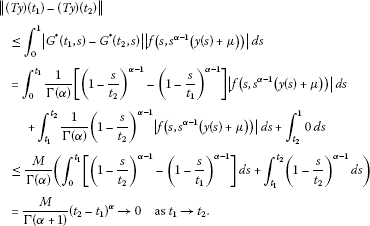According to Step 1-Step 3, the operator T is completely continuous in Ω. The proof is completed. □

Further, we have

Lemma 3.6 Suppose that$f\in C\left(\left[0,1\right]×R,R\right)$, and (2.4) and (2.6) holds, then, for any real number μ, the integral Equation (3.13) has at least one solution.

Proof We only need to show that the operator T is priori bounded. Let

$r=max\left\{1,\frac{{\int }_{0}^{1}{G}^{\ast }\left(s,s\right)a\left(s\right)\phantom{\rule{0.2em}{0ex}}ds+{\int }_{0}^{1}{G}^{\ast }\left(s,s\right)b\left(s\right)\phantom{\rule{0.2em}{0ex}}ds{|\mu |}^{p}}{1-{\int }_{0}^{1}{G}^{\ast }\left(s,s\right)b\left(s\right)\phantom{\rule{0.2em}{0ex}}ds}\right\}.$
(3.16)

Define a set $K\in \mathrm{\Omega }$ as follows

$K=\left\{y\in \mathrm{\Omega }|\parallel y\left(t\right)\parallel \le r\right\}.$
(3.17)

To show the existence of a fixed point of T by Lemma 2.3, we need to verify that the second possibility in Lemma 2.3 cannot happen.

In fact, assume that there exists $y\in \partial K$ with $\parallel y\left(t\right)\parallel =r$ and $\gamma \in \left(0,1\right)$ such that $y=\gamma Ty$. It follows that

$y\left(t\right)=\gamma |\left(Ty\right)\left(t\right)|=\gamma {\int }_{0}^{1}{G}^{\ast }\left(t,s\right)f\left(s,{s}^{\alpha -1}\left(y\left(s\right)+\mu \right)\right)\phantom{\rule{0.2em}{0ex}}ds,$

and

$\begin{array}{rl}\parallel y\left(t\right)\parallel & =\parallel \gamma {\int }_{0}^{1}{G}^{\ast }\left(t,s\right)f\left(s,{s}^{\alpha -1}\left(y\left(s\right)+\mu \right)\right)\phantom{\rule{0.2em}{0ex}}ds\parallel \\ \le \gamma {\int }_{0}^{1}{G}^{\ast }\left(s,s\right)|f\left(s,{s}^{\alpha -1}\left(y\left(s\right)+\mu \right)\right)|\phantom{\rule{0.2em}{0ex}}ds\\ <{\int }_{0}^{1}{G}^{\ast }\left(s,s\right)a\left(s\right)\phantom{\rule{0.2em}{0ex}}ds+{\int }_{0}^{1}{G}^{\ast }\left(s,s\right)b\left(s\right)\phantom{\rule{0.2em}{0ex}}ds{\parallel y\left(t\right)+\mu \parallel }^{p}\\ \le {\int }_{0}^{1}{G}^{\ast }\left(s,s\right)a\left(s\right)\phantom{\rule{0.2em}{0ex}}ds+{\int }_{0}^{1}{G}^{\ast }\left(s,s\right)b\left(s\right)\phantom{\rule{0.2em}{0ex}}ds{\parallel \mu \parallel }^{p}+{\int }_{0}^{1}{G}^{\ast }\left(s,s\right)b\left(s\right)\phantom{\rule{0.2em}{0ex}}ds{\parallel r\parallel }^{p}\\ \le {\int }_{0}^{1}{G}^{\ast }\left(s,s\right)a\left(s\right)\phantom{\rule{0.2em}{0ex}}ds+{\int }_{0}^{1}{G}^{\ast }\left(s,s\right)b\left(s\right)\phantom{\rule{0.2em}{0ex}}ds{\parallel \mu \parallel }^{p}+{\int }_{0}^{1}{G}^{\ast }\left(s,s\right)b\left(s\right)\phantom{\rule{0.2em}{0ex}}ds\parallel r\parallel \\ \le \parallel r\parallel .\end{array}$
(3.18)

Here we have the use of the inequality

${\left(a+b\right)}^{p}\le {a}^{p}+{b}^{p}\phantom{\rule{1em}{0ex}}\text{for}a,b\ge 0,0\le p\le 1.$

It is obvious that (3.18) contradicts our assumption that $\parallel y\left(t\right)\parallel =r$. Therefore, by Lemma 2.3, it follows that T has a fixed point $y\in \overline{K}$. Hence, the integral Equation (3.14) has at least a solution $y\left(t\right)$. The proof is completed. □

## 4 The proof of the main results

Now, we prove Theorem 2.1 by Lemma 3.4-3.5 and the intermediate value theorem.

Proof of Theorem 2.1 It is obvious that the right-hand side of (3.14) is continuously dependent on the parameter μ, so we need to find a μ such that $y\left(1\right)=0$, which implies that $u\left(1\right)=\mu$.

For any given real number μ, we rewrite (3.13) as follows:

${y}_{\mu }\left(t\right)={\int }_{0}^{1}{G}^{\ast }\left(t,s\right)f\left(s,{s}^{\alpha -1}\left({y}_{\mu }\left(s\right)+\mu \right)\right)\phantom{\rule{0.2em}{0ex}}ds,\phantom{\rule{1em}{0ex}}t\in \left[0,1\right].$
(4.1)

From (4.1), it suffices to show that there exists a μ such that

$L\left(\mu \right):={y}_{\mu }\left(1\right)={\int }_{0}^{1}{G}^{\ast }\left(1,s\right)f\left(s,{s}^{\alpha -1}\left({y}_{\mu }\left(s\right)+\mu \right)\right)\phantom{\rule{0.2em}{0ex}}ds.$
(4.2)

It is obvious that ${y}_{\mu }\left(1\right)$ is continuously dependent on the parameter μ. In order to prove that there exists a ${\mu }^{\ast }$ such that ${y}_{{\mu }^{\ast }}\left(1\right)=0$, we only need to show that ${lim}_{\mu \to \mathrm{\infty }}L\left(\mu \right)=\mathrm{\infty }$, and ${lim}_{\mu \to -\mathrm{\infty }}L\left(\mu \right)=-\mathrm{\infty }$.

Now, we show that ${lim}_{\mu \to \mathrm{\infty }}L\left(\mu \right)=\mathrm{\infty }$. On the contrary, we assume that ${\underline{lim}}_{\mu \to \mathrm{\infty }}L\left(\mu \right)<\mathrm{\infty }$. Then, there exists a sequence $\left\{{\mu }_{n}\right\}$, ${lim}_{n\to \mathrm{\infty }}{\mu }_{n}=\mathrm{\infty }$ such that ${lim}_{{\mu }_{n}\to \mathrm{\infty }}L\left({\mu }_{n}\right)<\mathrm{\infty }$, which implies that the sequence $\left\{L\left({\mu }_{n}\right)\right\}$ is bounded from above. Notice that the function $f\left(t,{t}^{\alpha -1}y\right)$ is continuous with respect to $t\in \left[0,1\right]$ and $y\in R$. We first claim that it is impossible to have

$f\left(t,{t}^{\alpha -1}\left({y}_{{\mu }_{n}}\left(t\right)+{\mu }_{n}\right)\right)\ge 0\phantom{\rule{1em}{0ex}}\text{for all}t\in \left[0,1\right]$
(4.3)

as ${\mu }_{n}$ is large enough. Indeed, assume that (4.3) is true. Then, by (4.1), we have

${y}_{{\mu }_{n}}\left(t\right)\ge 0$
(4.4)

for all $t\in \left[0,1\right]$. Thus, we get

$\underset{{\mu }_{n}\to \mathrm{\infty }}{lim}\left({y}_{{\mu }_{n}}\left(t\right)+{\mu }_{n}\right)=\mathrm{\infty }$
(4.5)

for all $t\in \left[0,1\right]$. Since we have assumed in (H) that

$\underset{u\to \mathrm{\infty }}{lim}f\left(t,{t}^{\alpha -1}u\right)=\mathrm{\infty },\phantom{\rule{1em}{0ex}}t\in \left(0,1\right),$
(4.6)

by (4.2), (4.5)-(4.6), we have

$\begin{array}{rcl}\underset{{\mu }_{n}\to \mathrm{\infty }}{lim}{y}_{{\mu }_{n}}\left(1\right)& =& \underset{{\mu }_{n}\to \mathrm{\infty }}{lim}{\int }_{0}^{1}{G}^{\ast }\left(1,s\right)f\left(s,{s}^{\alpha -1}\left({y}_{{\mu }_{n}}\left(s\right)+{\mu }_{n}\right)\right)\phantom{\rule{0.2em}{0ex}}ds\\ =& \underset{{\mu }_{n}\to \mathrm{\infty }}{lim}{\int }_{\frac{1}{4}}^{\frac{3}{4}}{G}^{\ast }\left(1,s\right)f\left(s,{s}^{\alpha -1}\left({y}_{{\mu }_{n}}\left(s\right)+{\mu }_{n}\right)\right)\phantom{\rule{0.2em}{0ex}}ds\\ =& \mathrm{\infty },\end{array}$
(4.7)

Now, for large ${\mu }_{n}$, we define

${I}_{n}=\left\{t\in \left[0,1\right]|f\left(t,{t}^{\alpha -1}\left({y}_{{\mu }_{n}}\left(t\right)+{\mu }_{n}\right)\right)<0\right\}.$

Then, ${I}_{n}$ is not empty.

Further, we divide the set ${I}_{n}$ into two sets ${\stackrel{˜}{I}}_{n}$ and ${\stackrel{ˆ}{I}}_{n}$ as follows:

${\stackrel{˜}{I}}_{n}=\left\{t\in {I}_{n}|{y}_{{\mu }_{n}}\left(t\right)+{\mu }_{n}>0\right\},\phantom{\rule{2em}{0ex}}{\stackrel{ˆ}{I}}_{n}=\left\{t\in {I}_{n}|{y}_{{\mu }_{n}}\left(t\right)+{\mu }_{n}\le 0\right\}.$

It is easy to know that ${\stackrel{˜}{I}}_{n}\cap {\stackrel{ˆ}{I}}_{n}=\varphi$, and ${\stackrel{˜}{I}}_{n}\cup {\stackrel{ˆ}{I}}_{n}={I}_{n}$, and we have from (H) that ${\stackrel{ˆ}{I}}_{n}$ is not empty.

From (H) again, the function $f\left(t,{t}^{\alpha -1}u\right)$ is bounded below by a constant for $t\in \left[0,1\right]$ and $u\in \left[0,\mathrm{\infty }\right)$. Thus, there exists a constant M (<0), independent of t and ${\mu }_{n}$, such that

$f\left(t,{t}^{\alpha -1}\left({y}_{{\mu }_{n}}\left(t\right)+{\mu }_{n}\right)\right)\ge M,\phantom{\rule{1em}{0ex}}t\in {\stackrel{˜}{I}}_{n}.$
(4.8)

Let

$\overline{m}\left({\mu }_{n}\right)=\underset{t\in {I}_{n}}{min}{y}_{{\mu }_{n}}\left(t\right).$

From the definitions of ${\stackrel{˜}{I}}_{n}$ and ${\stackrel{ˆ}{I}}_{n}$, we have

$\overline{m}\left({\mu }_{n}\right)=\underset{t\in {\stackrel{ˆ}{I}}_{n}}{min}{y}_{{\mu }_{n}}\left(t\right)=-{\parallel {y}_{{\mu }_{n}}\left(t\right)\parallel }_{{\stackrel{ˆ}{I}}_{n}},$

and it follows that $\overline{m}\left({\mu }_{n}\right)\to -\mathrm{\infty }$ as ${\mu }_{n}\to \mathrm{\infty }$ (since if $\overline{m}\left({\mu }_{n}\right)$ is bounded below by a constant as ${\mu }_{n}\to \mathrm{\infty }$, then (4.7) holds). Therefore, we can choose ${\mu }_{{n}_{1}}$ large enough so that

$\overline{m}\left({\mu }_{n}\right)
(4.9)

for $n>{n}_{1}$. From (H), (4.1), (4.8)-(4.9), and the definitions of ${\stackrel{˜}{I}}_{n}$ and ${\stackrel{ˆ}{I}}_{n}$, for any ${\mu }_{n}>{\mu }_{{n}_{1}}$, we have

$\begin{array}{rcl}{y}_{{\mu }_{n}}\left(t\right)& =& {\int }_{0}^{1}{G}^{\ast }\left(t,s\right)f\left(s,{s}^{\alpha -1}\left({y}_{{\mu }_{n}}\left(s\right)+{\mu }_{n}\right)\right)\phantom{\rule{0.2em}{0ex}}ds\\ \ge & {\int }_{{I}_{n}}{G}^{\ast }\left(s,s\right)f\left(s,{s}^{\alpha -1}\left({y}_{{\mu }_{n}}\left(s\right)+{\mu }_{n}\right)\right)\phantom{\rule{0.2em}{0ex}}ds\\ \ge & {\int }_{{\stackrel{˜}{I}}_{n}}{G}^{\ast }\left(s,s\right)f\left(s,{s}^{\alpha -1}\left({y}_{{\mu }_{n}}\left(s\right)+{\mu }_{n}\right)\right)\phantom{\rule{0.2em}{0ex}}ds\\ +{\int }_{{\stackrel{ˆ}{I}}_{n}}{G}^{\ast }\left(s,s\right)\left(-a\left(s\right)-b\left(s\right){|{y}_{{\mu }_{n}}\left(s\right)+{\mu }_{n}|}^{p}\right)\phantom{\rule{0.2em}{0ex}}ds\\ \ge & \left(M{\int }_{{\stackrel{˜}{I}}_{n}}{G}^{\ast }\left(s,s\right)\phantom{\rule{0.2em}{0ex}}ds-{\int }_{{\stackrel{ˆ}{I}}_{n}}{G}^{\ast }\left(s,s\right)a\left(s\right)\phantom{\rule{0.2em}{0ex}}ds\right)\\ -{\int }_{{\stackrel{ˆ}{I}}_{n}}{G}^{\ast }\left(s,s\right)b\left(s\right)\phantom{\rule{0.2em}{0ex}}ds{\parallel {y}_{{\mu }_{n}}\left(t\right)+{\mu }_{n}\parallel }^{p},\end{array}$

from which it follows that

$\begin{array}{rcl}{y}_{{\mu }_{n}}\left(t\right)& \ge & M{\int }_{0}^{1}{G}^{\ast }\left(s,s\right)\phantom{\rule{0.2em}{0ex}}ds-{\int }_{0}^{1}{G}^{\ast }\left(s,s\right)a\left(s\right)\phantom{\rule{0.2em}{0ex}}ds\\ -{\int }_{0}^{1}{G}^{\ast }\left(s,s\right)b\left(s\right)\phantom{\rule{0.2em}{0ex}}ds{\parallel {y}_{{\mu }_{n}}\left(t\right)\parallel }_{{I}_{n}}^{p}\\ \ge & M{\int }_{0}^{1}{G}^{\ast }\left(s,s\right)\phantom{\rule{0.2em}{0ex}}ds-{\int }_{0}^{1}{G}^{\ast }\left(s,s\right)a\left(s\right)\phantom{\rule{0.2em}{0ex}}ds\\ +{\int }_{0}^{1}{G}^{\ast }\left(s,s\right)b\left(s\right)\phantom{\rule{0.2em}{0ex}}ds\overline{m}\left({\mu }_{n}\right),\phantom{\rule{1em}{0ex}}t\in {I}_{n},\end{array}$

which implies that

$\overline{m}\left({\mu }_{n}\right)\ge \frac{M{\int }_{0}^{1}{G}^{\ast }\left(s,s\right)\phantom{\rule{0.2em}{0ex}}ds-{\int }_{0}^{1}{G}^{\ast }\left(s,s\right)a\left(s\right)\phantom{\rule{0.2em}{0ex}}ds}{1-{\int }_{0}^{1}{G}^{\ast }\left(s,s\right)b\left(s\right)\phantom{\rule{0.2em}{0ex}}ds}.$

Now, we have proved that ${lim}_{\mu \to \mathrm{\infty }}L\left(\mu \right)=\mathrm{\infty }$. By a similar method, we can prove that ${lim}_{\mu \to -\mathrm{\infty }}L\left(\mu \right)=-\mathrm{\infty }$. The detail is omitted.

Notice that $L\left(\mu \right)$ is continuous with respect to $\mu \in \left(-\mathrm{\infty },\mathrm{\infty }\right)$. It follows from the intermediate value theorem  that there exists a ${\mu }^{\ast }\in \left(-\mathrm{\infty },\mathrm{\infty }\right)$ such that $L\left({\mu }^{\ast }\right)=0$, that is $y\left(1\right)={y}_{{\mu }^{\ast }}\left(1\right)=0$, which satisfies the second boundary value condition of (1.2). The proof is completed. □

## 5 Examples

Example 5.1 Consider the following boundary value problem

$\left\{\begin{array}{c}{D}^{3/2}u\left(t\right)+{t}^{2}+\frac{u}{2}=0,\phantom{\rule{1em}{0ex}}t\in \left[0,1\right],\hfill \\ u\left(0\right)=0,\phantom{\rule{2em}{0ex}}u\left(1\right)={2}^{\frac{1}{2}}u\left(\frac{1}{2}\right),\hfill \end{array}$
(5.1)

where

$\alpha =3/2,\phantom{\rule{2em}{0ex}}\eta =\frac{1}{2},\phantom{\rule{2em}{0ex}}{2}^{\frac{1}{2}}\cdot {\left(\frac{1}{2}\right)}^{\frac{3}{2}-1}=1,$

and

$f\left(t,u\right)={t}^{2}+\frac{u}{2},\phantom{\rule{2em}{0ex}}f\left(t,{t}^{\frac{1}{2}}u\right)={t}^{2}+{t}^{\frac{1}{2}}\frac{u}{2},\phantom{\rule{2em}{0ex}}b\left(t\right)=\frac{{t}^{\frac{1}{2}}}{2}.$

It is easy to show that

$\underset{u\to ±\mathrm{\infty }}{lim}f\left(t,{t}^{\frac{1}{2}}u\right)=±\mathrm{\infty },\phantom{\rule{1em}{0ex}}t\in \left(0,1\right),$

and

$\begin{array}{rcl}{\int }_{0}^{1}{G}^{\ast }\left(s,s\right)b\left(s\right)\phantom{\rule{0.2em}{0ex}}ds& \le & \frac{1}{2}\cdot \frac{1}{\mathrm{\Gamma }\left(\frac{3}{2}\right)\left(1-{\left(\frac{1}{2}\right)}^{\frac{1}{2}}\right)}{\int }_{0}^{1}{\left(1-s\right)}^{\frac{1}{2}}{s}^{\frac{1}{2}}\phantom{\rule{0.2em}{0ex}}ds\\ =& \frac{1}{2}\cdot \frac{1}{\mathrm{\Gamma }\left(\frac{3}{2}\right)\left(1-{\left(\frac{1}{2}\right)}^{\frac{1}{2}}\right)}\cdot \frac{\mathrm{\Gamma }\left(\frac{3}{2}\right)\mathrm{\Gamma }\left(\frac{3}{2}\right)}{\mathrm{\Gamma }\left(3\right)}\\ \approx & 0.756<1.\end{array}$

Thus, the conditions of Theorem 2.1 are satisfied. Therefore, the problem (5.1) has at least a nontrivial solution.

## Author’s contributions

Each of the authors, ZO and GL contributed to each part of this study equally and read and approved the final version of the mnanuscript.

## References

1. Kilbas AA, Srivastava HM, Trujillo JJ: Theory and Applications of Fractional Differential Equations. Elsevier, Amstadam; 2006.

2. Bakhani A, Daftardar-Gejji V: Existence of positive solutions of nonlinear fractional differential equations. J. Math. Anal. Appl. 2003, 278: 434-442. 10.1016/S0022-247X(02)00716-3

3. Bakhani A, Daftardar-Gejji V: Positive solutions of a system of non-autonomous fractional differential equations. J. Math. Anal. Appl. 2005, 302: 56-64. 10.1016/j.jmaa.2004.08.007

4. Li CF, Luo XN, Zhou Y: Existence of positive solutions of the boundary value problem for nonlinear fractional differential equations. Comput. Math. Appl. 2010, 59: 1363-1375. 10.1016/j.camwa.2009.06.029

5. Bai CZ: Positive solutions for nonlinear fractional differential equations with coefficient that changes sign. Nonlinear Anal. 2006, 64: 677-685. 10.1016/j.na.2005.04.047

6. Delbosco D: Fractional calculus and function spaces. J. Fract. Calc. 1994, 6: 45-53.

7. Jiang DQ, Yuan CJ: The positive properties of the Green function for Dirichlet-type boundary value problems of nonlinear fractional differential equations and its application. Nonlinear Anal. 2010, 72: 710-719. 10.1016/j.na.2009.07.012

8. Kaufmann ER, Mboumi E: Positive solutions of a boundary value problem for a nonlocal fractional differential equations. Electron. J. Qual. Theory Differ. Equ. 2008, 3: 1-11.

9. Jafari H, Gejji VD: Positive solutions fractional nonlinear boundary value problems using Adomian decomposition method. Appl. Math. Comput. 2006, 180: 700-706. 10.1016/j.amc.2006.01.007

10. Podlubny I: Fractional Differential Equations. Academic Press, New York; 1993.

11. Liao KR, Li ZY: Mathematical Analysis. Higher Education Press, Beijing; 1986. in Chinese

12. Belmekki M, Benchohra M: Existence results for fractional order semilinear functional differential equations with nondense domain. Nonlinear Anal. 2010, 72: 925-932. 10.1016/j.na.2009.07.034

13. Benchohra M, Hamani S, Ntouyas SK: Boundary value problems for differential equations with fractional order and nonlocal conditions. Nonlinear Anal. 2009, 71: 2391-2396. 10.1016/j.na.2009.01.073

14. EI-Shahed M: Positive solutions for boundary value problem of nonlinear fractional differential equations. Abstr. Appl. Anal. 2007., 2007:

15. Kosmatov N: Integral equations and initial value problems for nonlinear differential equations of fractional order. Nonlinear Anal. 2009, 70: 2521-2529. 10.1016/j.na.2008.03.037

16. Agarmal RP, Meehan M, O’Regan D: Fixed Point Theory and Applications. Cambridge University Press, Cambridge; 2001.

17. Agarwal RP, Zhou Y, He Y: Existence of fractional neutral functional differential equations. Comput. Math. Appl. 2010, 59: 1095-1110. 10.1016/j.camwa.2009.05.010

18. Liang SH, Zhang JH: Positive solutions for boundary value problems of nonlinear fractional differential equation. Nonlinear Anal. 2009, 71: 5545-5550. 10.1016/j.na.2009.04.045

19. Zhang SQ: Positive solutions for boundary value problems of nonlinear fractional differential equations. Electron. J. Differ. Equ. 2006, 2006: 1-12.

20. Gejji VD: Positive solutions of a system of non-autonomous fractional differential equations. J. Math. Anal. Appl. 2005, 302: 56-64. 10.1016/j.jmaa.2004.08.007

21. Lakshmikantham V: Theory of fractional function differential equations. Nonlinear Anal. 2008, 69: 3337-3343. 10.1016/j.na.2007.09.025

22. Lakshmikantham V, Vatsala AS: Basic theory of fractional differential equations. Nonlinear Anal. 2008, 69: 2677-2682. 10.1016/j.na.2007.08.042

23. Lakshmikantham V, Leela S, Vasundhara Devi J: Theory of Fractional Dynamic Systems. Cambridge Scientific Publishers, Cambridge; 2009.

24. Zhou Y, Jiao F, Li J: Existence and uniqueness of fractional neutral differential equations with infinite delay. Nonlinear Anal. 2009, 71: 3249-3256. 10.1016/j.na.2009.01.202

25. Bai ZB: On positive solutions of a nonlocal fractional boundary value problem. Nonlinear Anal. 2010, 72: 916-924. 10.1016/j.na.2009.07.033

26. Bai ZB, Lu HS: Positive solutions for boundary value problem of nonlinear fractional differential equation. J. Math. Anal. Appl. 2005, 311: 495-505. 10.1016/j.jmaa.2005.02.052

## Acknowledgements

Supported partially by China Postdoctoral Science Foundation under Grant No.20110491280 and the Subject Lead Foundation of University of South China No. 2007XQD13.

## Author information

Authors

### Corresponding author

Correspondence to Zigen Ouyang.

### Competing interests

The authors declare that they have no competing interests.

## Rights and permissions

Reprints and Permissions

Ouyang, Z., Li, G. Existence of the solutions for a class of nonlinear fractional order three-point boundary value problems with resonance. Bound Value Probl 2012, 68 (2012). https://doi.org/10.1186/1687-2770-2012-68

• Accepted:

• Published:

• DOI: https://doi.org/10.1186/1687-2770-2012-68

### Keywords

• Banach Space
• Real Number
• Integral Equation
• Green Function
• Fractional Order